Question

Four long parallel wires each carry 2.0 A in the same direction. They are parallel to...

Four long parallel wires each carry 2.0 A in the same direction. They are parallel to the z-axis, and they pass through the corners of a square of side 4.0 cm positioned in the x-y plane. What net magnetic field does one of the wires experience due to the other wires?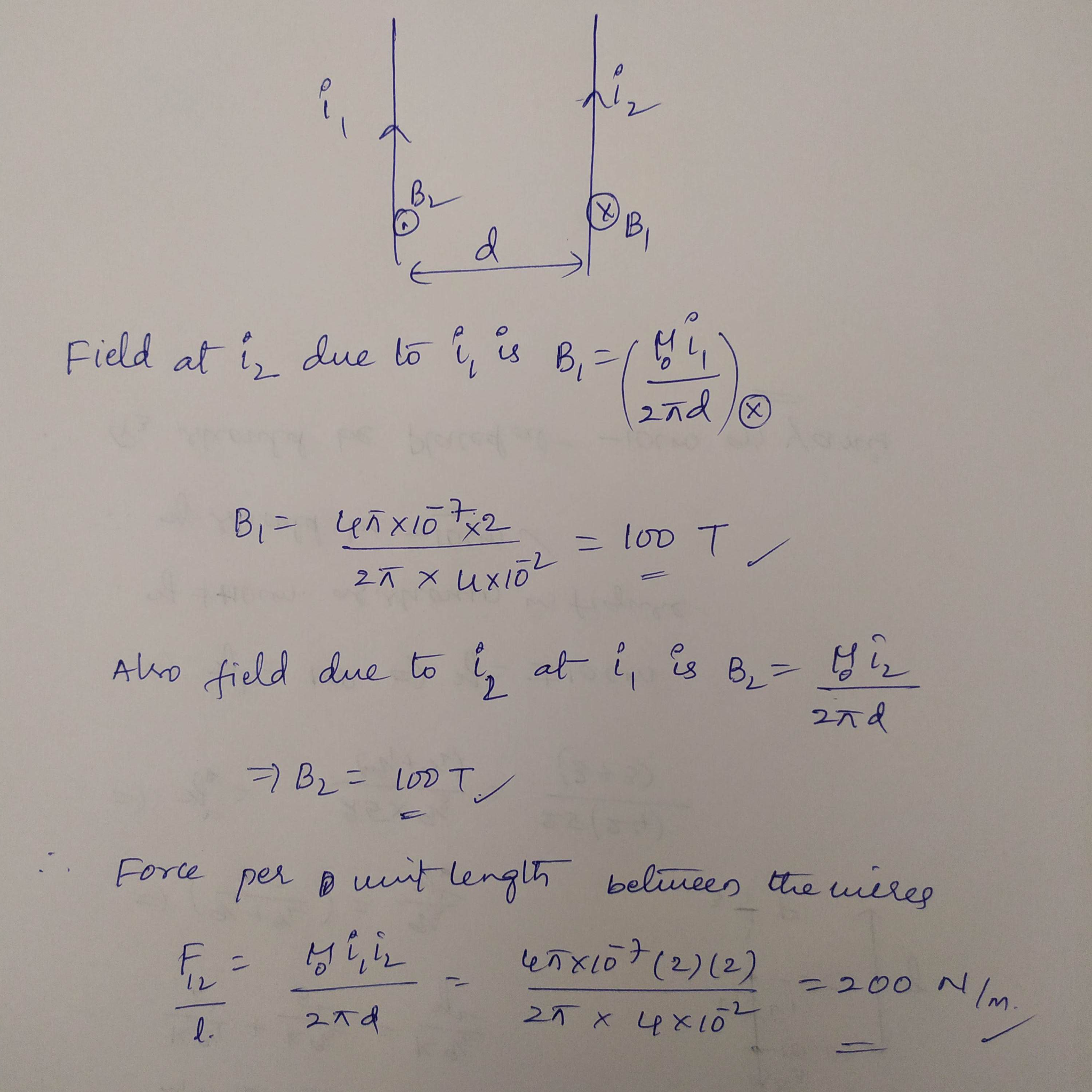Earn Coins

Coins can be redeemed for fabulous gifts.

Similar Homework Help Questions
• Four long parallel wires each carry a 2.0 A current in the same direction. The wires...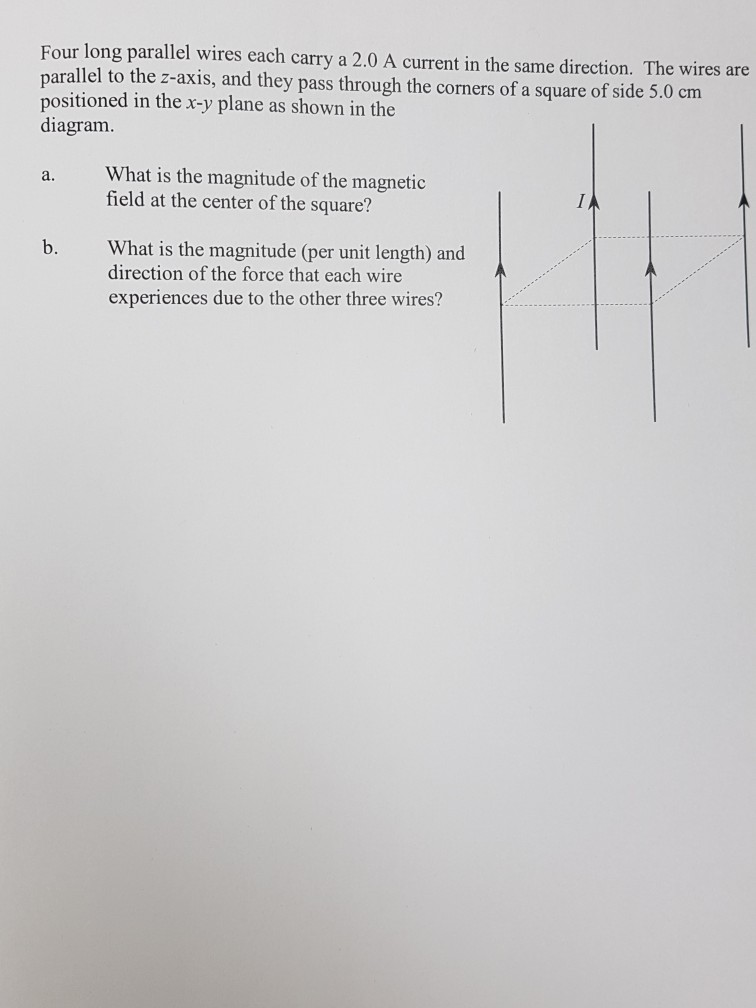Four long parallel wires each carry a 2.0 A current in the same direction. The wires are parallel to the z-axis, and they pass through the corners of a square of side 5.0 cm in the x-y plane as shown in the diagram. What is the magnitude of the magnetic field at the center of the square? a. b. What is the magnitude (per unit length) and direction of the force that each wire experiences due to the other three...

• ch.28 12

Four long parallel wires each carry 2.0 A in the same direction. They are parallel to the z-axis, and they pass through the corners of a square of side 4.0 cmpositioned in the x-y plane. What magnetic field does one of the wires experience due to the other wires?

• Four long parallel wires pass through the corners of a square with side 0.62 m. All...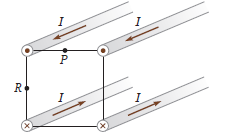Four long parallel wires pass through the corners of a square with side 0.62 m. All four wires carry the same magnitude of current I = 24.1 A in the directions indicated. Find the magnetic field at point P, the midpoint of the top side of the square. Four long parallel wires pass through the corners of a square with side 0.62 m. All four wires carry the same magnitude of current I = 24.1 A in the directions indicated....

• 9. Four wires are running parallel at the corners of a square. The wires run parallel to the x ax...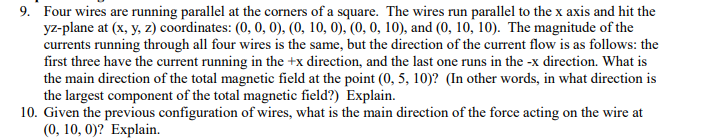9. Four wires are running parallel at the corners of a square. The wires run parallel to the x axis and hit the yz-plane at (x, y, z) coordinates: (0, 0, 0), (0, 10, 0), (0, 0, 10), and (0, 10, 10). The magnitude of the currents running through all four wires is the same, but the direction of the current flow is as follows: the first three have the current running in the +x direction, and the last one...

• Four long straight parallel wires are oriented perpendicular to the x-y plane and carry currents I1,...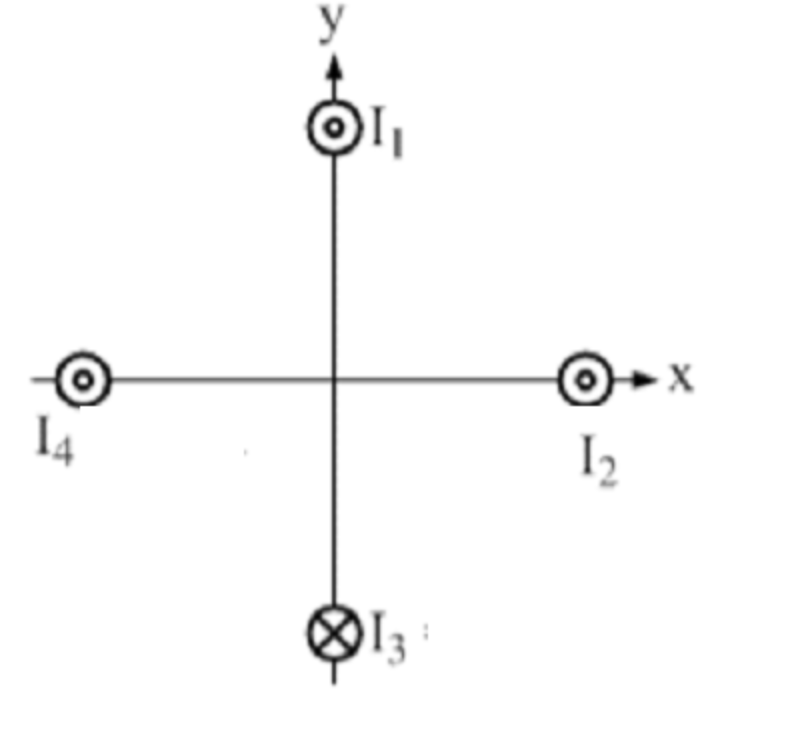Four long straight parallel wires are oriented perpendicular to the x-y plane and carry currents I1, I2, I3 and I4. The magnitudes of the currents are all identical and equal to 4.015 A. Current I3 is into the x-y plane (i.e. into the screen), whereas each of the other three currents are all out of the x-y plane(i.e. out of the screen) as shown below. Each of the wires is a distance of 2.987 cm from the origin as measured...

• Four long straight parallel wires are oriented perpendicular to the x-y plane and carry currents I1,...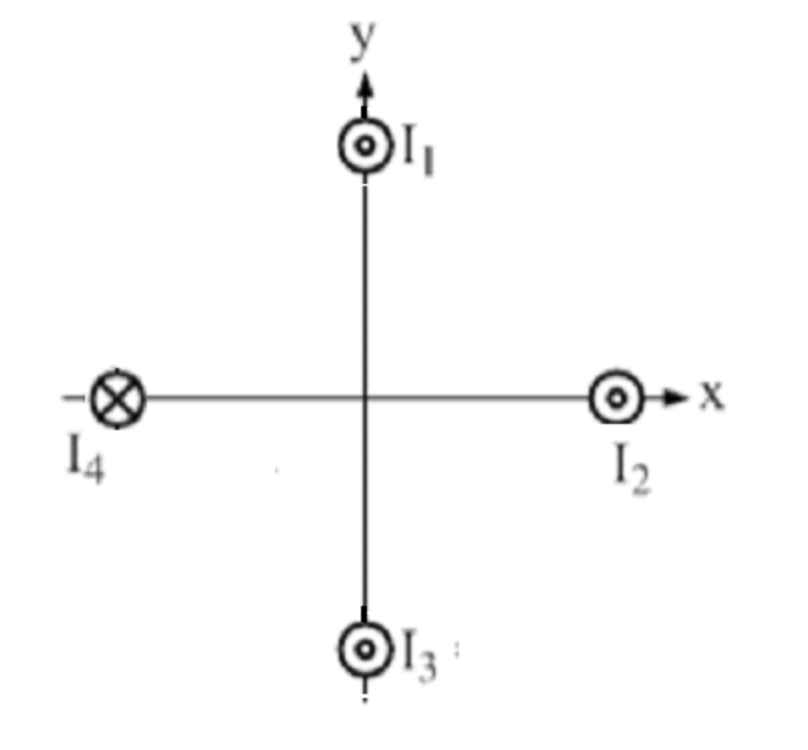Four long straight parallel wires are oriented perpendicular to the x-y plane and carry currents I1, I2, I3 and I4. The magnitudes of the currents are all identical and equal to 8.475 A. Current I4 is into the x-y plane (i.e. into the screen), whereas each of the other three currents are all out of the x-y plane(i.e. out of the screen) as shown below. Each of the wires is a distance of 6.105 cm from the origin as measured...

• Four long parallel wires each carry a current of 1.0 A. The current direction is out...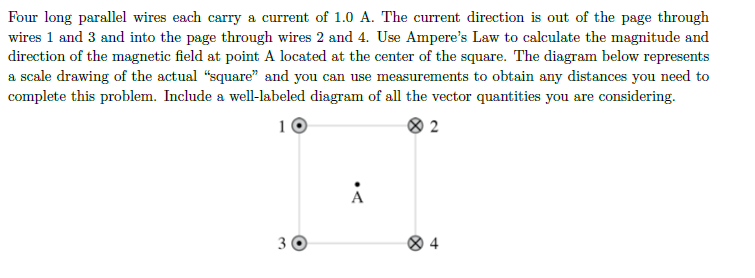Four long parallel wires each carry a current of 1.0 A. The current direction is out of the page through wires 1 and 3 and into the page through wires 2 and 4. Use Ampere's Law to calculate the magnitude and direction of the magnetic field at point A located at the center of the square. The diagram below represents a scale drawing of the actual "square" and you can use measurements to obtain any distances you need to complete...

• The figure below shows two long, parallel wires in cross-section, and the currents they carry are...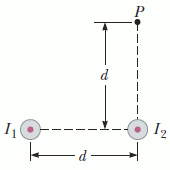The figure below shows two long, parallel wires in cross-section, and the currents they carry are directed out of the page. The left wire carries a current of I1 = 2.96 A, and the right carries a current of I2 = 3.45 A. The two wires are a distance d = 20.0 cm apart. Point P is the same distance d above the right wire. Assume the +x-direction is to the right, and the +y-direction is upward. A cross-sectional end...

• Two long, parallel wires carry currents of I1 = 4.80 A and I2 = 1.80 A...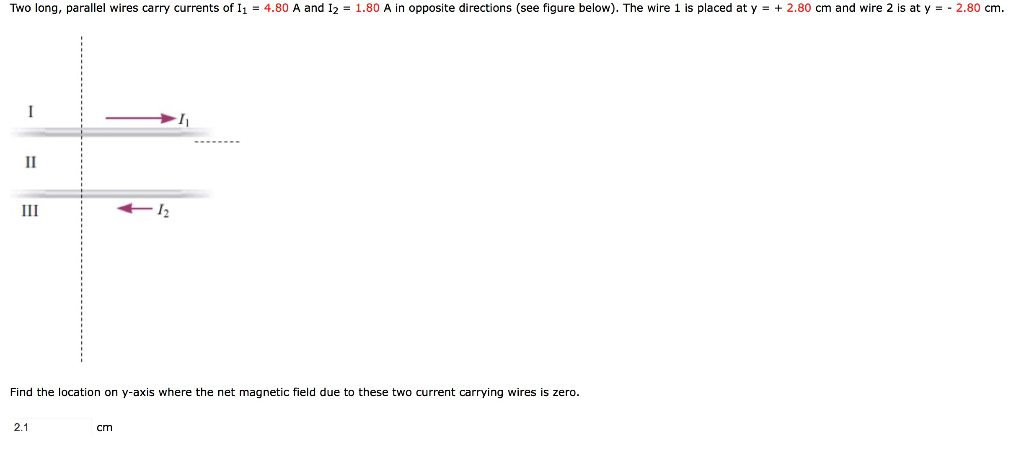Two long, parallel wires carry currents of I1 = 4.80 A and I2 = 1.80 A in opposite directions (see figure below). The wire 1 is placed at y = + 2.80 cm and wire 2 is at y = - 2.80 cm. Find the location on y-axis where the net magnetic field due to these two current carrying wires is zero. ** my answer was wrong and I can't figure out how to correctly solve this** Two long, parallel...

• Two long thin parallel wires 13.0 cm apart carry 24-Acurrents in the same direction. Part A:...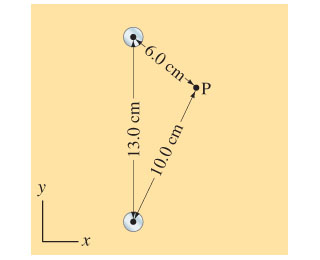Two long thin parallel wires 13.0 cm apart carry 24-Acurrents in the same direction. Part A: Determine the magnitude of the magnetic field vector at a point 10.0 cm from one wire and 6.0 cm from the other (Figure 1) . Part B: Determine the direction of the magnetic field vector at that point. degrees, measured counterclockwise from the positive x axis